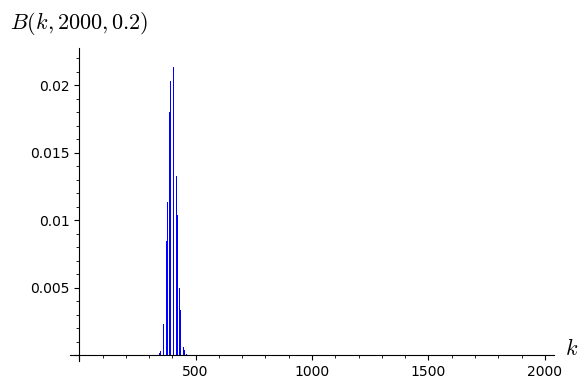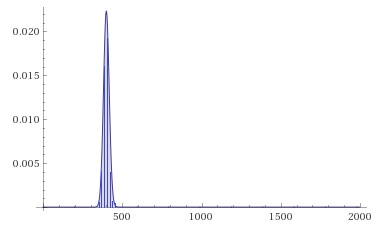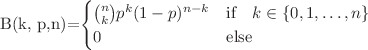2021-07-13 23:53:38 +0200 received badge ● Famous Question (source) 2021-05-07 19:40:01 +0200 received badge ● Famous Question (source) 2021-03-10 12:32:19 +0200 received badge ● Good Question (source) 2021-01-02 16:55:43 +0200 received badge ● Notable Question (source) 2020-12-29 20:44:43 +0200 received badge ● Notable Question (source) 2020-11-12 09:00:18 +0200 received badge ● Popular Question (source) 2020-10-21 18:20:48 +0200 commented answer How to get access of a single solution from a bunch of solutions Ah, nice ;) 2020-10-21 14:12:04 +0200 asked a question How to get access of a single solution from a bunch of solutions I do not use the thing for longer time, so I had a question. If I say a,b,c,d,e,x = var('a b c d e x') f(x)=a*x^4+b*x^3+c*x^2+d*x+e f1(x)=diff(f(x),x) f2(x)=diff(f(x),x,2) sols = solve([f(0)==-2, f(2)==0, f1(4)==0, f(4)==8, f2(2)==0], a,b,c,d,e) print(sols)  I get  [ [a == (3/16), b == (-41/16), c == (87/8), d == -12, e == -2] ]  How can I have these single value separately? That means: I want to get an output f(x) = 3/16*x^4 -41/16*x^3 +87/8*x^2 -12*x -2 (which I can copy and paste). 2020-10-21 12:56:23 +0200 commented answer Plot the solution of a system of equations TYVM. Helps me good. ;) 2020-10-21 12:07:25 +0200 asked a question Plot the solution of a system of equations With a,b,c,d,e,x = var('a b c d e x') f(x)=a*x^4+b*x^3+c*x^2+d*x+e f1(x)=diff(f(x),x) f2(x)=diff(f(x),x,2) solve([f(0)==-3, f(2)==0, f2(4)==0, f1(6)==0, f(6)==4], a,b,c,d,e)  I get [[a == (-7/432), b == (43/216), c == (-5/6), d == (5/2), e == -3]] How can I plot f(x) with these values? 2020-09-21 11:29:27 +0200 received badge ● Nice Question (source) 2020-09-21 11:29:21 +0200 received badge ● Self-Learner (source) 2020-08-31 14:45:20 +0200 received badge ● Popular Question (source) 2020-04-06 13:54:57 +0200 commented answer Syntax to define a Layout of a Plot I have to use your code in the kind Layout = dict(frame=False, axes_labels=['$k$','$B(k,{},{})$'.format(n,float(p))]) bar_chart([binom_dist.pmf(x) for x in range(n)], **Layout) But this way it works. 2020-04-06 13:50:54 +0200 commented answer How to get a nice bar chart? Very good, too. :() 2020-04-06 13:50:37 +0200 commented answer How to get a nice bar chart? Very good. And yes, I note that as well. ;) 2020-04-06 13:02:48 +0200 commented answer Syntax to define a Layout of a Plot Ah, very good! ;) 2020-04-06 12:39:04 +0200 commented answer How to plot the Binomial Distribution Nice... ;) 2020-04-06 10:54:56 +0200 asked a question How to get a nice bar chart? I havereset() import scipy.stats n = 2000 p = 0.2 binom_dist = scipy.stats.binom(n,p) bar_chart([binom_dist.pmf(x) for x in range(n)], axes_labels=['$k$','$B(k,{},{})$'.format(n,float(p))])  How could I create something nicer, like2020-04-06 10:37:32 +0200 asked a question Syntax to define a Layout of a Plot Just for interest: I have reset() import scipy.stats n = 2000 p = 0.2 binom_dist = scipy.stats.binom(n,p) bar_chart([binom_dist.pmf(x) for x in range(n)], frame=True, axes_labels=['$k$','$B(k,{},{})$'.format(n,float(p))])  How could outsource the part like Layout = frame=True, axes_labels=['$k$','$B(k,{},{})$'.format(n,float(p))]? And then bar_chart([binom_dist.pmf(x) for x in range(n)], Layout)  2020-04-05 19:47:23 +0200 asked a question How to plot the Binomial Distribution Hey there, is there a macro for? 2020-01-17 01:36:32 +0200 received badge ● Self-Learner (source) 2020-01-09 20:26:44 +0200 commented question Is there an inline command, that cleans the cache? Probably it is reset() 2020-01-09 20:21:11 +0200 edited answer Introduction: A shortcut for the compiling of SageTeX-files with arara If you use arara, known as "The cool TeX-automatisation Tool", written by @PauloCereda, you can set compilation instructions into your tex-document, i.e. % arara: pdflatex % arara: biber ..... and then only one run arara example.tex is needed. In TeXLive arara is installed by default. You can also integrate arara into your TeX editor. There is no arara-rule for Sage in the main set of arara-rules, but it has been written: See the original post arara: sage - A Sage(math)-rule for arara It is sagetex.yaml, which works for Windows-users and for Linux-users: !config # SageTeX-Rule for arara. # # Dear Windows-users, please check the paths # pathToBashExecutive and pathToSageStartfile # due to your Sage-installation! # identifier: sagetex name: SageTeX authors: - TeXnician (Author) - cis (Idea) arguments: [] commands: - name: A SageTeX Rule for arara command: > @{ pathToBashExecutive = "C:\\Programme\\SageMath8.9\\runtime\\bin\\bash"; pathToSageStartfile = "C:/Programme/SageMath8.9/runtime/opt/sagemath-8.9/sage"; pathOfCurrentWorkingFolder = currentFile().getParent(); theWindowsCommand = getCommand(pathToBashExecutive, "-l", pathToSageStartfile, "-c", "os.chdir('" + pathOfCurrentWorkingFolder + "'); load('" + getBasename(currentFile()) + ".sagetex.sage')"); return isWindows(theWindowsCommand, getCommand("sage", getBasename(file) + ".sagetex.sage")); }  Let's test an example.tex % arara: pdflatex % arara: sagetex % arara: pdflatex \documentclass{report} \usepackage{sagetex} \begin{document} \sage{2^1234} \end{document}  2020-01-09 19:28:26 +0200 commented answer Correct way to get an exact number of decimal digits (after the point) @dsejas OK, I understand. Seems your code has a little mistake. Suggestion: def NumPrint(x, n): d = floor(log(abs(x), 10)) + 1 return N(x, digits=n+d)#, d print NumPrint(-100.25,2) print NumPrint(1/14,7) print NumPrint(0.0123, 4)  2020-01-07 15:27:04 +0200 commented question Max Min and Min Max Your real question is probably: "How do I start / install Sage?" Your other question: "How do I calculate the maximum of ...?" assumes the functioning of the first problem; and should then be in a separate thread; in particular, an example should be given for that second question. 2020-01-05 21:52:08 +0200 marked best answer SageTeX-Output: Possible simplification sought I created a minimal example for SageTeX: x = 420 # Denominator MyRange = 222 M = [] for n in range (1,MyRange): if x == denominator(n/x): M.append('$' + latex(n/x).replace(' ','') + '$') else: M.append('$' + '\\frac{' + "{}".format(n) + '}{' + "{}".format(x) + '}=' + latex(n/x).replace(' ','') + '$') MyOut = ', '.join(M) print(MyOut)  This gives:It works! Just for interest, I wonder: does the 2nd else-output M.append('$' + '\\frac{' + "{}".format(n) + '}{' + "{}".format(x) + '}=' + latex(n/x).replace(' ','') + '$') really have to be so complicated or is there an easier way? €dit: My SageTeX-MWE is: \documentclass{article} \usepackage{amsmath, amssymb, amsfonts} \usepackage{sagetex} \begin{document} \section{In} \begin{sageblock} x = 420 # Denominator MyRange = 222 M = [] for n in range (1,MyRange): if x == denominator(n/x): M.append('$' + latex(n/x).replace(' ','') + '$') else: M.append('$' + '\\frac{' + "{}".format(n) + '}{' + "{}".format(x) + '}=' + latex(n/x).replace(' ','') + '$') MyOut = ', '.join(M) #print MyOut \end{sageblock} \section{Out} \baselineskip16pt \sagestr{MyOut} \end{document}